# How to view the Vout of opamp on LTSpice

#### djsfantasi

Joined Apr 11, 2010
8,792
Run the simulation. When it completes, with the mouse hover over “v0”. The onscreen mouse will change to a probe icon. Click. It’s values will be added to the plot pane.

#### ericgibbs

Joined Jan 29, 2010
17,174
hi 123,
Post your LTS asc file, so that we can edit/add as required, to give the result you are asking.
E

#### LvW

Joined Jun 13, 2013
1,622
You want a "scope equivalent"?
What do you see on a scope? A voltage as a function of time , right?
However, in your simulation profile I see an AC input voltage (frequency domain).
I think, it is logical to use an input signal as a function of time and to perform a TRAN analysis in the time domain to meet your needs.

#### Alec_t

Joined Sep 17, 2013
13,337
Your sim is set up by the .DC command to do a DC analysis. What you want instead is a .TRANS analysis, with V1 set, for example, as a SINE generator.

#### quique123

Joined May 15, 2015
405
Here it is. Ive tried the .tran command but I get 0V for the Vout node and 0 A for the power source as SINE 1V amplitude

#### Attachments

• 811 bytes Views: 3

#### eetech00

Joined Jun 8, 2013
3,418
Here it is. Ive tried the .tran command but I get 0V for the Vout node and 0 A for the power source as SINE 1V amplitude
Specify a frequency in the sine voltage source. It should look like this:

SINE(0 1 1k)

Where 1k=1000 Hz or whatever frequency you want.

#### BobTPH

Joined Jun 5, 2013
6,310
I think you might benefit from following an LTSPICE tutorial.

Bob

#### Audioguru

Joined Dec 20, 2007
11,248
The opamp is not powered. The Sim program assumes that it has a supply that is at least plus and minus 12VDC.
If the power supply does not include the negative 12VDC then the output of the opamp clips and rectifies the signal.

#### quique123

Joined May 15, 2015
405
Thanks! I think ive sort of got it: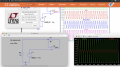I did get something cleared in my mind, which is why I wanted to do this. Since I first learned about opamps, I wanted to understand how it works. I spent weeks reviewing videos and asking on forums as to how it worked and I started piece together how it worked. I thought I had it and left it at that and moved over to other arduino and rpi projects. But now I see that I had the wrong idea. I thought it got a small ac signal in and Vout would vary until the difference between both terminals was as close to zero as possible (per the definition), and then it stabilized. But according to this its still an oscillating Vout. So it keeps "varying". I guess im confusing ac varying signal with a somehow unstable signal.

#### LvW

Joined Jun 13, 2013
1,622
I thought it got a small ac signal in and Vout would vary until the difference between both terminals was as close to zero as possible (per the definition), and then it stabilized. But according to this its still an oscillating Vout. So it keeps "varying". I guess im confusing ac varying signal with a somehow unstable signal.
Well, I think most of the problems you have faced were caused by some confusion regarding the different analyses.
Example: In the 1st picture you have selecteds an AC source - and your analysis command was "DC analysis".

The most important analyses are

1.) DC analysis: Vary one DC voltage source (or current source) and display the answer of the circuit: DC voltages and currents at/through the various nodes.

2.) AC anaylysis: Vary the frequency of a AC source (constant amplitude) and watch how the magnitudes and phases of the various node voltages (or branch currents) are changing with frequency.

3.) TRAN analysis: Use an input signal as a function of time with a specified waveform (pulse, triangle, sinus) and watch the various node voltages resp. currents as a function of time (like a virtual oscilloscope)

#### Bordodynov

Joined May 20, 2015
3,038
I think that your misunderstanding is due to the fact that textbooks impose abstraction with virtual zero. Without explaining why this can be considered. In a real (and ideal) circuit, a small differential voltage difference at the input (0) is maintained by negative feedback. If the feedback is positive, then it is not. I made the gain changes from 100 to 100 Meg = 1.e+8. Moreover, the greater the gain, the smaller the voltage difference at the input of the amplifier. For an ideal amplifier, the gain tends to infinity and the voltage difference tends to zero. In a real amplifier, there is still a zero offset.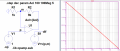#### Audioguru

Joined Dec 20, 2007
11,248
An opamp has an extremely high voltage gain but negative feedback reduces the gain so that the input voltages are almost the same.

#### quique123

Joined May 15, 2015
405
ok thanks, i think what I wanted was obscured by my lack of understanding and confusion. Ok so the first thing I wanted to do was model a capacitor circuit which showed how the current flows into the LED sub-circuit and the capacitor sub-circuit, lighting up the LED and charging the capacitor and then show how when the power source is removed, the LED slowly turns off as the capacitor discharges its current into the LED-sub-circuit. So I was imagining current flowing around due to voltage difference stored in the Cap.

Then I thought of one of my favorite examples, an oscillator, and I envisioned it how the left transistor charges the left cap and then it switches to the other side, lighting up the different LEDs each time.

Finally I thought of the opamp, and for some reason I thought there would be some charging up (i dont know why because there are no caps). I dont know what I was thinking. I guess I thought I might visualize the phrase "an opamp does whatever it has to do to make the input terminal differential equal to 0)" and this made me think of an exponential as in the case of the charging capacitor. But here is the image of my opamp circuit showing V(in) as +1V and the resulting and amplified V(out) as 10V due to my R choice and V(f) for feedback terminal equal to V(in) at 1V: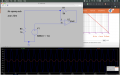Im still not sure what I wanted to visualize, perhaps its the current flows as mentioned above...ill try that...ok so I did and I can see currents through V(out) and R(1). I(out) decreases as V(out) increases, but at the same time I(R) increases. Can someone help me understand why? Im very visual about these things unfortunately.

If V(out) must make V(f) = 1V of V(in), then V(out) increases to 10V according to its gain. But then some of that gets fed back into the V(f). Why if I(out) is so little, even negative, why is there so much current going thru R1? Its not going thru R3 to GND because R3 current is also just as low as I(out). Im confused...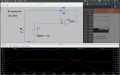#### crutschow

Joined Mar 14, 2008
31,544

#### quique123

Joined May 15, 2015
405
Ok I was measuring I(R1) instead of I(U1ut). Apparently there is a difference, so ok, Id like to understand 2 things:

1. I understand that V(in) is 1V and so Vout is 10x and they should be in sync because the signal is going into the non-inverting terminal.

1.A I(U1ut) and I(R1) are in sync, makes sense. But why are I(R1) and I(R3) out of sync?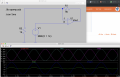This is my initial drawing: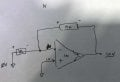and this is my completed drawing. As I worked the circuit using Ohms Law, I first wrote down the voltages, then determined direction of current flow and finally calculared current values. However this was all based on the fact that Vout is 10V. So it seems that that output which is calculated using the ideal opamp gain formula must be given first and only THEN can you calculate the rest. It makes it seem like the 2 calculations (the current flows and Vout calc) are unrelated, or rather you must set Vout first in order to get the other calculations: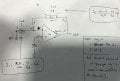#### crutschow

Joined Mar 14, 2008
31,544
I was measuring I(R1) instead of I(U1ut). Apparently there is a difference
Only in the polarity of the current direction.

The polarity of the current through a resistor depends its orientation, which is arbitrary (you can reverse it by selecting the resistor with the Move command, and using CTRL R to rotate it).
If you put the cursor over the resistor after a simulation, an arrow will appear that shows the positive direction of current in the resistor.

In my simulation, I orientated the resistors so that their arrows were both pointed towards the op amp output, thus all the currents (resistors and op amp output) are positive at the same time (since current into the op amp output is positive), and thus all current-direction polarities are opposite to the op amp output voltage polarity.

#### quique123

Joined May 15, 2015
405
Thanks, I reoriented my R and all is fine.

Could I ask you to address my issue from Post#18 starting at:

"This is my initial drawing:"

which has to do with the analysis of the opamp circuit?